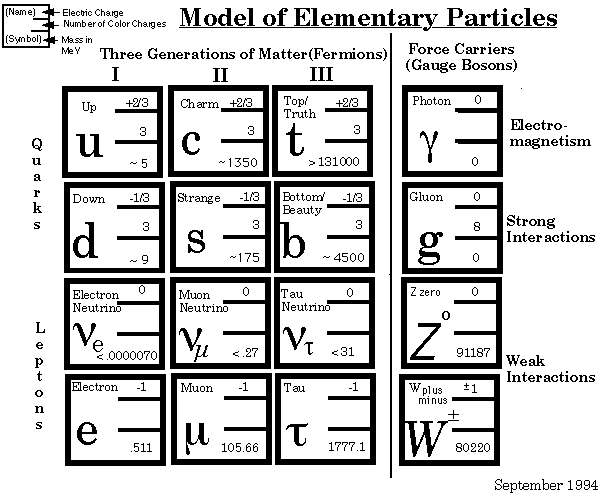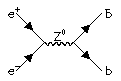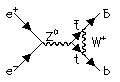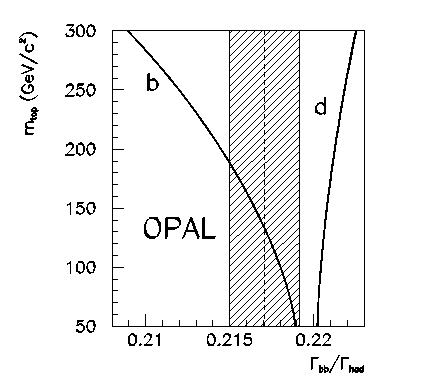# Precision ElectroWeak Physics

A unified theory of the weak and electromagnetic interactions was developed in the late 1960s by Glashow, Weinberg and Salam. A distinctive feature of this unification, which was not present in either of the older theories, was the introduction of a massive neutral gauge boson, the Z0. The Z boson was first observed at CERN in 1983, and one of the principal objectives of LEP is to produce Z Bosons copiously, and to test the properties of the Z against the predictions of the Standard Model.

The particles of the Standard Model are shown in this chart:The theory predicts precisely how the Z should couple to each of the different types of particles. For example, the production of b-quarks in the decay of the Z proceeds through a mechanism like this:.

This is a particularly important case, because the formation of b-quarks is influenced by more complicated diagrams involving the very heavy top t-quark, like this:.

Although the t-quark is heavier than the Z Boson, we are still indirectly sensitive to its existence.

The most powerful method for tagging b-quarks depends on the fact that the hadrons containing b-quarks have lifetimes around 1 picosecond, and therefore travel typically a few millimetres before decaying. Such decays can be identified using the silicon microvertex detector, and this has been the basis of the work at Cambridge. If you click here you will see an example of a Z decay to b quarks. If you zoom in you can see clear secondary decay vertices. By comparing the numbers of events in which one or two such secondary vertices are seen, we can infer the tagging efficiency from the data, and thus make a precise measurement of the rate of Z decays to b-quarks.

The OPAL measurement for the fraction of Z decays yielding b-quarks is 0.2171+-0.0021+-0.0021, where the first error is statistical, and the second systematic. The figure below shows how the OPAL measurement agrees with the Standard Model prediction. The measurement with its error is shown by the shaded band, and the theory predictions for b- and d-quarks (which depend on the mass of the t-quark) are shown as curves. You can read the whole paper by clicking here.